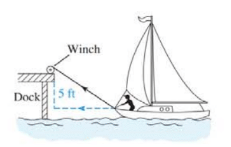Chapter 11.4, Problem 32E### Mathematical Applications for the ...

11th Edition
Ronald J. Harshbarger + 1 other
ISBN: 9781305108042

#### Solutions

Chapter
Section### Mathematical Applications for the ...

11th Edition
Ronald J. Harshbarger + 1 other
ISBN: 9781305108042
Textbook Problem

# Boat docking Suppose that a boat is being pulled toward a dock by a winch that is 5 ft above the level of the boat deck, as shown in the figure. If the winch is pulling the cable at a rate of 3 ft/min, at what rate is the boat approaching the dock when it is 12 ft from the dock?To determine

To calculate: The rate at which the boat is approaching the dock when it is 12 ft from dock if the boat is being pulled toward a dock by a winch that is 5 ft. above the level of the boat.

Explanation

Given Information:

Boat is 12 ft from dock if the boat is being pulled toward a dock by a winch that is 5 ft. above the level of the boat as shown in the provided figure,

And, the winch is pulling the cable at the rate of 3 ft/min.

Formula used:

According to the Pythagoras theorem,

a2+b2=c2

Where, a,b,c are the sides of the triangle.

Calculation:

Consider the provided figure,

Now, x be the length of the cable and y be the horizontal distance from the dock to the boat, then, according to the Pythagoras theorem,

x2=y2+25 …… (1)

As the boat is 12 ft from the dock, substitute 12 for y to get,

x2=144+25x2=169x=13

Now, differentiate both sides of equation (1) with respect to

### Still sussing out bartleby?

Check out a sample textbook solution.

See a sample solution

#### The Solution to Your Study Problems

Bartleby provides explanations to thousands of textbook problems written by our experts, many with advanced degrees!

Get Started

#### Find more solutions based on key concepts